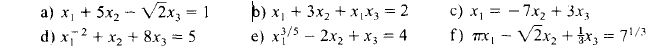# Linear equations

## Homework Statement

i am doing homework about linear equations, i am just asking if i am ok, whith my selection
the question is: from the next equations, which ones are line equations, in x1,x2 and x3?

## Homework Equations[/B]

## The Attempt at a Solution

the next are line; a,c,f
the next arent line; b,d,e
correct me if i am wrong please[/B]

member 587159
Looks right.

SammyS
Staff Emeritus
Homework Helper
Gold Member

## Homework Statement

i am doing homework about linear equations, i am just asking if i am ok, whith my selection
the question is: from the next equations, which ones are line equations, in x1,x2 and x3?

## Homework Equations

View attachment 100077 [/B]

## The Attempt at a Solution

:[/B]
the next are line; a,c,f
the next arent line; b,d,e
correct me if i am wrong please
The terminology is "linear equation".